# Abstract approximation theory

Jump to: navigation, search

Classical approximation theory deals with the approximation of real-valued functions defined on real intervals by certain basic functions like ordinary or trigonometric polynomials (cf. also Trigonometric polynomial), splines (see Spline approximation), etc. An approximation may be obtained through coincidence on a given subset of the domain (interpolation and trigonometric interpolation) or through more general constructive or non-constructive methods. The quality of an approximation is usually evaluated by a measure for the deviation of two functions (see Approximation of functions, measure of). These approaches may be suitably transferred to more general settings.

## Interpolation.

A general formulation of an interpolation problem in normed spaces is due to L. Asimow [a2]. Letbe a closed linear subspace in a normed linear space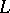. Given a bounded closed convex neighbourhoodof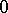inand a bounded closed convex set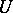containing, their polars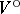and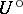in the adjoint spaceof(cf. also (the editorial comments to) Polar), one defines onthe functionals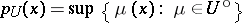and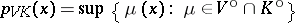. For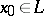one looks for an element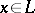satisfying:

1)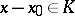;

2a)(exact solution); respectively,

2b)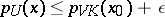(approximate solution). In the classical situation,stands for a space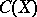of continuous functions on a compact spaceendowed with the supremum norm, andfor all functions vanishing on a compact subset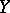of. Further,is the class of functions used for the interpolation. Condition 1) means thatandcoincide on, whereas 2a) (respectively, 2b)) impose further restrictions on the interpolating element. Classical results on this problem include the Urysohn and Rudin–Carleson theorems for function spaces. The general case requires technical conditions involving the dual space of.

## Approximation by linear operators.

Approximation schemes may often be modelled by sequences (or generalized sequences) of linear operators (see Approximation of functions, linear methods). For a sequence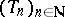of positive linear operators on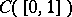, Korovkin's theorem [a4] states that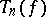converges uniformly tofor every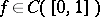whenever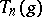converges to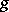for the three test functions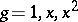(cf. also Korovkin theorems). This result was subsequently generalized to different sets of test functionsand various topological spacesreplacing the interval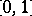(cf. also Korovkin-type approximation theory). Classical examples include the Bernstein operators (see Bernstein polynomials) and the Fejér sums (cf. also Fejér sum), which provide approximation schemes by polynomials and trigonometric polynomials, respectively. Further generalizations investigate the convergence of certain classes of linear operators on various domains, such as positive operators on topological vector lattices, contractive operators on normed spaces, multiplicative operators on Banach algebras, monotone operators on set-valued functions, monotone operators with certain restricting properties on spaces of stochastic processes, etc. (cf. [a1] for a recent survey). Typically, for a subsetof the domain, one defines the Korovkin closureofas the set of all elements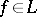such thatwhenever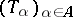is an equicontinuous generalized sequence (cf. Equicontinuity) in the restricted class of operators such that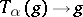holds for all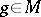. The main problem is to describe the Korovkin closure as explicitly as possible.

A very general setting allowing a unified approach to most of the above-mentioned cases is given by locally convex cones [a3], structures that generalize locally convex ordered vector spaces (cf. Locally convex space; Semi-ordered space) in the sense that scalar multiplication is only defined for non-negative real numbers; neither the existence of negatives nor the validity of the cancellation law is required. In spite of their generality, locally convex cones allow a rich duality theory, including Hahn–Banach-type extension and separation theorems for continuous linear functionals. Various restrictions on classes of linear operator may be taken care of by proper choices for the domains and their topologies alone, and Korovkin closures may be characterized in terms of the dual cone.

A complete description of Korovkin closures is available for positive linear operators on weighted spaces of continuous functions [a5], [a6]: Given a locally compact spaceand an upward-directed familyof non-negative upper semi-continuous real-valued weight functions on, one considers the space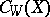of all continuous real-valued functionsonsuch that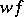vanishes at infinity for all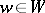. Endowed with the semi-norms(cf. Semi-norm),is a locally convex space. (The case, for example, leads towith the topology of uniform convergence; if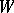consists of the characteristic functions of all compact subsets of, thenwith the topology of compact convergence.) For a subsetof, a functionis contained in the Korovkin closure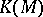if and only if for allsuch thatfor someone (and therefore both) of the following two equivalent conditions hold:

a)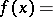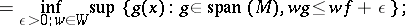b) for every finite positive regular Borel measureonand every,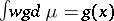for allimplies. If, then b) shows that the vector sublattice generated byis dense in. In this way the preceding criterion leads to versions of the classical Stone–Weierstrass theorem.